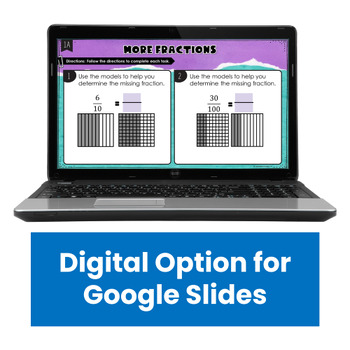4th
Subjects
Standards
Resource Type
Formats Included
• PDF
•Google Apps™
PagesThe Teacher-Author indicated this resource includes assets from Google Workspace (e.g. docs, slides, etc.).

Also included in

1. Do you need a way to easily differentiate your math practice or homework to your students? Need a way to provide differentiated math support for your students at home? This set of differentiated math printables for 4th grade is just what you need!Note: This resource now contains a Google Classroom a
\$9.00
\$18.00
Save \$9.00
2. Do you want your math stations and guided math centers done for the year? This 4th grade guided math bundle is the only resource you will need to have your math centers done for the year!Need 3rd or 5th Grade Math Centers?Click here to see the 3rd Grade Guided Math Mega Bundle.Click here to see the
\$95.00
\$168.50
Save \$73.50

Description

This resource contains three printables for each standard in the Number and Operations: Fractions domain of the 4th Grade Common Core standards.

There are 9 skills covered in this resource, differentiated at three levels, for a total of 27 printables.

Want more 4th Grade Differentiated Math Skill Sheets?

Here are the skills represented in this resource (remember there are three levels per skill):

1. Equivalent Fractions (4.NF.1)

2. Comparing Fractions (4.NF.2)

3. Adding and Subtracting Fractions (4.NF.3)

4. Decomposing Fractions (4.NF.3)

5. Adding and Subtracting Mixed Numbers (4.NF.3)

6. Multiplying Fractions and Whole Numbers (4.NF.4)

7. Adding Fractions with Denominators of 10 and 100 (4.NF.5)

8. Converting Fractions with Denominators of 10 and 100 to Decimals and Vice Versa (4.NF.6)

9. Comparing Decimals (4.NF.7)

The printables are differentiated by the following levels:

LLevel 1 (Below Grade Level): These printables provide support for the student to complete a grade-level problem.

Level 3 (Above Grade Level): These printables provide no additional support and also include problems that are more challenging.

The printables contain 100% different problems among the levels. This means that the teacher can use all three levels with the students, upping the levels as the students proceed through mastery of the standards.

These differentiated skill sheets could be used for class work, homework, or even assessments. These are ideal for differentiating for special education students, English Language Learners, and/or gifted students.

More 4th Grade Math Activities and Resources

Holiday and Seasonal Math Centers and Printables

Total Pages
Included
Teaching Duration
N/A
Report this Resource to TpT
Reported resources will be reviewed by our team. Report this resource to let us know if this resource violates TpT’s content guidelines.

Standards

to see state-specific standards (only available in the US).
Compare two decimals to hundredths by reasoning about their size. Recognize that comparisons are valid only when the two decimals refer to the same whole. Record the results of comparisons with the symbols >, =, or <, and justify the conclusions, e.g., by using a visual model.
Use decimal notation for fractions with denominators 10 or 100. For example, rewrite 0.62 as 62/100; describe a length as 0.62 meters; locate 0.62 on a number line diagram.
Express a fraction with denominator 10 as an equivalent fraction with denominator 100, and use this technique to add two fractions with respective denominators 10 and 100. For example, express 3/10 as 30/100, and add 3/10 + 4/100 = 34/100.
Apply and extend previous understandings of multiplication to multiply a fraction by a whole number.
Understand a fraction 𝘢/𝘣 with 𝘢 > 1 as a sum of fractions 1/𝘣.